# Dragging motion for pile of blocks

Jump to: navigation, search
This article discusses a scenario/arrangement whose statics/dynamics/kinematics can be understood using the ideas of classical mechanics.
View other mechanics scenarios

## The two blocks scenario

Consider one block placed on top of another. The blocks are cuboidal with a horizontal surface of contact. Suppose the lower block is$A$ and the upper block is$B$, and the lower block is resting on a fixed floor. Suppose the coefficients of friction are:

•$\mu_{s1}$ is the limiting coefficient of static friction between$A$ and the floor.
•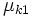$\mu_{k1}$ is the coefficient of kinetic friction between$A$ and the floor.
•$\mu_{s2}$ is the limiting coefficient of static friction between$A$ and$B$.
•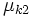$\mu_{k2}$ is the coefficient of kinetic friction between$A$ and$B$.

We assume that there are no external forces in the vertical direction, other than the gravitational and normal force.

## Normal force and gravitational force

### On the upper block

KEY FORCE CONCEPT (ACTION-REACTION): Just because two forces are equal in magnitude and opposite in direction does not imply that they form an action-reaction pair in the sense of Newton's third law of motion. An action-reaction pair is a pair occurs between two bodies that exert forces on each other, not for a pair of forces both acting on the same body. The normal force on the upper block and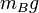$m_Bg$ (the gravitational force) are equal and opposite but do not form an action reaction pair.

If we denote by$N_{AB}$ the normal force between the blocks, then$N_{AB}$ acts upward on the upper block. The gravitational force$m_Bg$ acts downward. Since there is no net acceleration in the vertical direction, we get: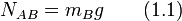$\! N_{AB} = m_Bg \qquad (1.1)$

### On the lower block

If we denote by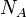$N_A$ the normal force between the lower block and the floor, we obtain, by Newton's first law, that: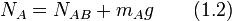$N_A = N_{AB} + m_Ag \qquad (1.2)$

Plugging in (1) into (2) we get: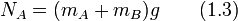$N_A = (m_A + m_B)g \qquad (1.3)$

Intuitively, the weight borne by the floor is the total of the weights of both blocks.

## Case of force on lower block

Suppose an external horizontal force$F$ is applied on the lower block. We determine the conditions under which both blocks start moving together, the two blocks start moving but not together, and neither block moves.

### Summary of cases

Verbal description of case Lower bound on$F$ for this case Upper bound on$F$ for this case Acceleration of$A$ Acceleration of$B$
Neither block moves 0$\! g[\mu_{s1}(m_A + m_B)]$ 0 0
Both blocks move together with the same acceleration$\! g[\mu_{s1}(m_A + m_B)]$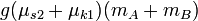$g(\mu_{s2} + \mu_{k1})(m_A + m_B)$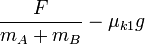$\frac{F}{m_A + m_B} - \mu_{k1}g$$\frac{F}{m_A + m_B} - \mu_{k1}g$
The blocks move with different accelerations$\frac{F}{m_A + m_B} - \mu_{k1}g$ infinite$\frac{1}{m_A}\left[F - g(\mu_{k1}(m_A + m_B) + \mu_{k2}m_B)\right]$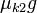$\mu_{k2}g$

### Situation where neither block moves

Denote by$f_{s1}$ the force of static friction between$A$ and the floor, and by$f_{s2}$ the force of static friction between$A$ and$B$.

We get the following equation in the horizontal direction: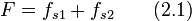$F = f_{s1} + f_{s2} \qquad (2.1)$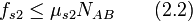$f_{s2} \le \mu_{s2} N_{AB} \qquad (2.2)$

On the other hand, the horizontal component of the force diagram of$B$ gives:$f_{s2} = 0 \qquad (2.3)$

Plugging in (1.3) and (2.2), we get:$f_{s1} \le \mu_{s1} (m_A + m_B)g \qquad (2.4)$

Plug (2.3) and (2.4) in (2.1) and obtain: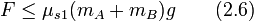$F \le \mu_{s1}(m_A + m_B)g \qquad (2.6)$

Thus, the maximum possible value of external force at which the system does not start accelerating is: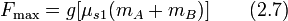$F_{\max} = g[\mu_{s1}(m_A + m_B)] \qquad (2.7)$

ANSWER FORMAT CHECK: For these kinds of situations, the critical values of external forces are always of the form$g$ times a homogeneous linear polynomial involving the masses, with coefficients drawn from various possible friction coefficient.

### Situation where blocks move together as a system

Suppose both blocks have an acceleration of$a$ in the direction of the external applied force$F$. Denote by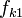$f_{k1}$ the force of kinetic friction between$A$ and the floor, and by$f_{s2}$ the force of static friction between$A$ and$B$ (the friction is static because the blocks move together so there is no slipping between surfaces). We get: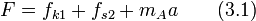$F = f_{k1} + f_{s2} + m_Aa \qquad (3.1)$

Also:$\! f_{k1} = \mu_{k1}N_A \qquad (3.2)$$\! f_{s2} \le \mu_{s2}N_{AB} \qquad (3.3)$

and finally, the horizontal force component on$B$ gives:$\! f_{s2} = m_Ba \qquad (3.4)$

Plug in (3.2) and (3.4) into (3.1) and get: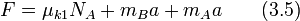$F = \mu_{k1}N_A + m_Ba + m_Aa \qquad (3.5)$

Plug in (1.3) in here to get:$F = \mu_{k1}(m_A + m_B)g + (m_A + m_B)a \qquad (3.6)$

Rearranging, we obtain: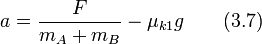$\! a = \frac{F}{m_A + m_B} - \mu_{k1}g \qquad (3.7)$

INTUITIVE CHECK: The value of acceleration is the same as what we would get if we assumed a single block of mass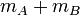$m_A + m_B$ with coefficient of kinetic friction$\mu_{k1}$ with the floor.

Also, we note by plugging in (3.3) into (3.4) that: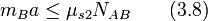$m_Ba \le \mu_{s2}N_{AB} \qquad (3.8)$

Plugging in (3.7) and (1.1) into (3.8) we get: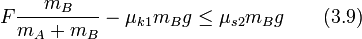$F \frac{m_B}{m_A + m_B} - \mu_{k1}m_Bg \le \mu_{s2}m_Bg \qquad (3.9)$

which rearranges to:$F \le (\mu_{s2} + \mu_{k1})(m_A + m_B)g \qquad (3.10)$

We already know from (2.7) a lower bound on$F$, so we get, combined, that:$\mu_{s1}(m_A + m_B)g \le F \le (\mu_{s2} + \mu_{k1})(m_A + m_B)g \qquad (3.11)$

### Situation where blocks move at separate accelerations

Suppose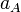$a_A$ is the acceleration of the lower block and$a_B$ is the acceleration of the upper block. Denote by$f_{k1}$ the force of kinetic friction between$A$ and the floor and by$f_{k2}$ the force of kinetic friction between$B$ and the floor. We get: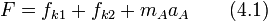$F = f_{k1} + f_{k2} + m_Aa_A \qquad (4.1)$

Also:$f_{k1} = \mu_{k1}N_A \qquad (4.2)$

and$f_{k2} = \mu_{k2} N_{AB} \qquad (4.3)$

The horizontal force component for$B$ gives:$f_{k2} = m_Ba_B \qquad (4.4)$

Plugging in (4.2) and (4.3) into (4.1), we get:$F = \mu_{k1}N_A + \mu_{k2}N_{AB} + m_Aa_A \qquad (4.5)$

Plugging in the values of$N_A$ and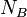$N_B$ from (1.1) and (1.3), we get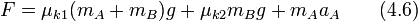$F = \mu_{k1}(m_A + m_B)g + \mu_{k2}m_Bg + m_Aa_A \qquad (4.6)$

This rearranges to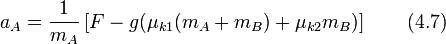$a_A = \frac{1}{m_A}\left[F - g(\mu_{k1}(m_A + m_B) + \mu_{k2}m_B)\right] \qquad (4.7)$

Plugging in (4.3) and (1.1) into (4.4), we get:$\mu_{k2}m_Bg = m_Ba_B \qquad (4.8)$

This rearranges to: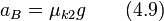$\! a_B = \mu_{k2}g \qquad (4.9)$

Finally, the constraint on$F$ is that: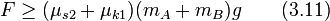$F \ge (\mu_{s2} + \mu_{k1})(m_A + m_B)g \qquad (3.11)$